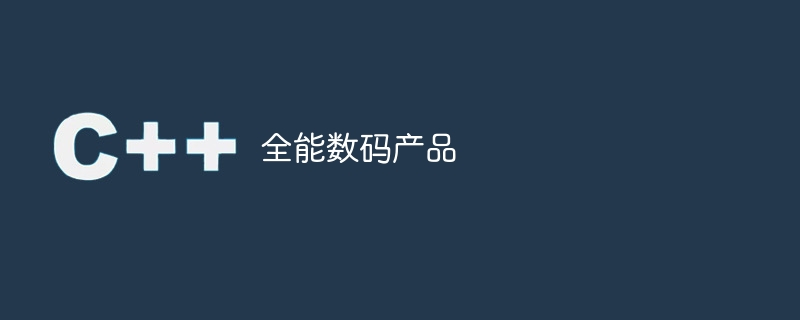# 全能数码产品WBOY 转载
2023-09-19 13:09:10 600浏览```Given Number: 7254
Result obtained: Yes, the condition is true
```

```Given Number: 6952
Result obtained: Yes, the condition is true
```

## 方法

• 第 1 步 - 迭代循环以检查该数字的所有因子对。

• 第二步 − 对于因子的每个部分，我们将创建一个包含原始数字和两个因子的字符串。

• 第三步 - 使用sort()函数对形成的字符串进行排序。

• 第 4 步 - 现在我们将创建另一个字符串“123456789”

• 第 5 步 - 比较两个字符串，如果相同则返回 true。

### 示例

```#include <bits/stdc++.h>
using namespace std;

// this function checks whether the given string consist of pandigital numbers
bool Is_Pandigital_product (string Original_string) {
if ( Original_string.length() != 9 ) {
return false;
}
char c[Original_string.length()];
strcpy(c, Original_string.c_str());
sort(c, c + Original_string.length());
string new_string = c;
if(new_string.compare("123456789") == 0) // comparing both the strings
{
return true;
} else {
return true;
}
}
bool PandigitalProduct_1_9(int num)
// This function iterates over a loop till Sqrt(n) and searches for factors of given input.
// for each factor, this loop calls for Is_Pandigital_product function
{
for (int Iterator = 1; Iterator * Iterator <= num; Iterator++)
if (num % Iterator == 0 && Is_Pandigital_product(to_string(num) + to_string(Iterator) + to_string(num / Iterator)))
return true; //Returns true if found pandigital number
return false;
}
int main() {
int num = 1234;
if (PandigitalProduct_1_9(num) == true)
cout << "yes the number " << num << " is a pandigital product";
else
cout << "no the number " << num <<" is not a pandigital product";
return 0;
}
```

### 输出

```yes the number 1234 is a pandigital product
```